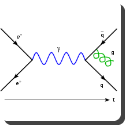Thursday , 18 August 2022

# What is broken symmetry?For spontaneous symmetry breaking to occur, there must be a system in which there are several equally likely outcomes. The system as a whole is therefore symmetric with respect to these outcomes (if we consider any two outcomes, the probability is the same). However, if the system is sampled (i.e. if the system is actually used or interacted with in any way), a specific outcome must occur. Though we know the system as a whole is symmetric, we also see that it is never encountered with this symmetry, only in one specific state. Because one of the outcomes is always found with probability 1, and the others with probability 0, they are no longer symmetric.

Hence, the symmetry is said to be spontaneously broken in that theory. Nevertheless, the fact that each outcome is equally likely is a reflection of the underlying symmetry, which is thus often dubbed “hidden symmetry”, and has crucial formal consequences, such as the presence of Nambu-Goldstone bosons.

## Kariamanikkam Srinivasa Krishnan Biography

Name: Kariamanikkam Srinivasa Krishnan FRS known as C. R. Rao Born: 4 December 1898 Watrap, …